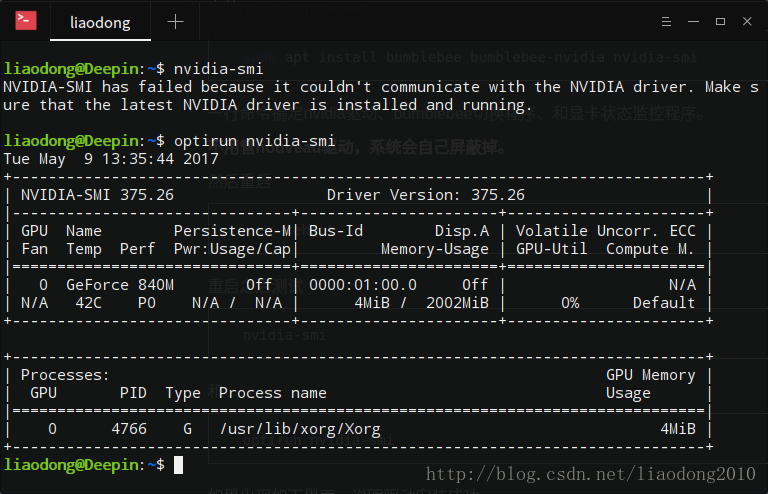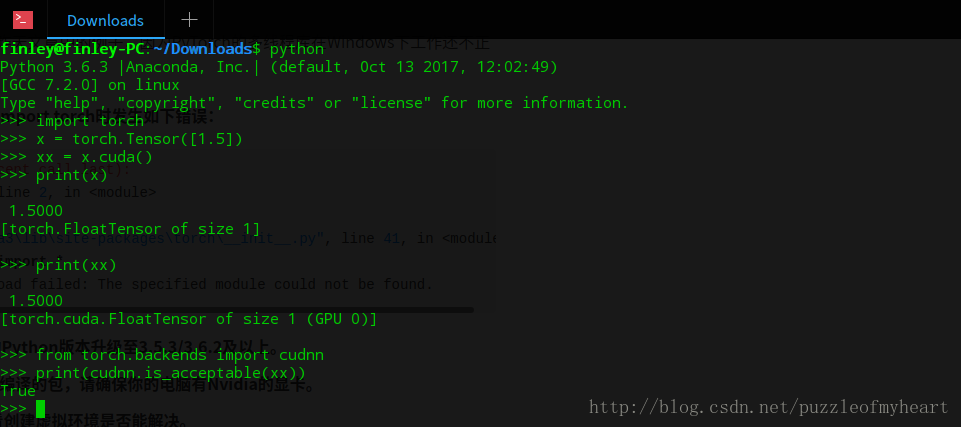• https://www.jiqizhixin.com/articles/2019-06-02-4 ***万字综述，核心开发者全面解读PyTorch内部机制 https://www.jiqizhixin.com/articles/2019-06-18-10 ***PyTorch代码调试利器: 自动print每行代码的... ...
https://www.jiqizhixin.com/articles/2019-06-02-4   ***万字综述，核心开发者全面解读PyTorch内部机制

https://www.jiqizhixin.com/articles/2019-06-18-10   ***PyTorch代码调试利器: 自动print每行代码的Tensor信息


展开全文• Pytroch深度学习分布式训练，Distributed training of Deep Learning models with PyTorch。
• 对一维信号，通常采取的方法有两种，第一，直接对其做一维卷积，第二，反映到时频图像上，这就变成了图像识别，此前一直都在利用keras搭建网络，最近学了pytroch搭建cnn的方法，进行一下代码的尝试。所用数据为经典...
• PyTroch学习笔记一、与numpy有关1、tensor与numpy转换 一、与numpy有关 1、tensor与numpy转换 tensor初始化 a = torch.ones(5) print(a) 输出 tensor([1., 1., 1., 1., 1.]) 转换为numpy b = a.numpy() print(b) ...
PyTroch学习笔记一、基本概念类1、与numpy有关1、tensor与numpy转换2、ToTensor：3、维度扩充4、torch求解梯度二、内置函数2.1 transforms模块2.2 torch.squeeze() 和torch.unsqueeze()的用法2.3 ModuleList 和 Sequential: 区别和使用场景2.4 nn 与 nn.functional 有什么区别2.5 nn.Module三、保存和加载模型1、加载预训练模型2、保存和加载自己训练过的模型及参数四、数据读取4.1 torch.utils.data.Dataset()4.1.1 __getitem__(self, index)4.2 torch.utils.data.DataLoader()4.2.1 collate_fn()五、Tensorboard5.15.n 注意事项六、踩坑记录6.1 复制相关clone()detach()使用分析100、出现的error1、RuntimeError: Expected 4-dimensional input for 4-dimensional weight 64 3 3, but got 3-dimensional input of size [3, 224, 224] instead2、明明写着有显存剩余，但还是会报错：CUDA out of memory. Tried to allocate 14.13 MiB (GPU 0; 6.00 GiB total capacity; 356.92 MiB already allocated; 3.99 GiB free; 6.58 MiB cached)3、Runtime error(59):device-side assert triggered at XXX4. 当某个函数一直出现没有 “xx”属性
一、基本概念类
1、与numpy有关
1、tensor与numpy转换
PS:转换后的tensor与numpy指向同一地址，所以，对一方的值改变另一方也随之改变
（1）tensor初始化，与numpy的使用方法相同
a = torch.ones(5)
print(a)

输出
tensor([1., 1., 1., 1., 1.])

（2）tensor to numpy
b = a.numpy()
print(b)

输出
[1. 1. 1. 1. 1.]

a.add_(1)
print(a)	# tensor([2., 2., 2., 2., 2.])
print(b)	# [2. 2. 2. 2. 2.]

（3）numpy to tensor
import numpy as np
a = np.ones(5)
b = torch.from_numpy(a)
np.add(a, 1, out=a)
c = torch.add
print(a)
print(b)

输出
[2. 2. 2. 2. 2.]
tensor([2., 2., 2., 2., 2.], dtype=torch.float64)

除chartensor外所有tensor都可以转换为numpy
2、ToTensor：
convert a PIL image to tensor (H,W,C) in range [0,255] to a torch.Tensor(C,H,W) in the range [0.0,1.0]
tensor = transforms.ToTensor(image)

注意：当然这个图像可以是Image读取的；也可以使用opencv读取。而后者需要将读取的图像颜色通道需要从BGR转换为RGB格式。不知道为什么，虽然使用的效果相近，但两则的转换结果并不是完全一样的。
3、维度扩充
大多数模型的输入维度都是(B,C,W,H)，但我们使用单张图片测试模型效果时，只有(C,W,H)三个通道的数据，此时，则需要扩充数据维度，代码如下：
"""
src-(C,W,H)
des-(B,C,W,H)
"""
des= Variable(torch.unsqueeze(src, dim=0).float(), requires_grad=False)

PS：也可按照2.2节的方式进行维度扩充
4、torch求解梯度
1、requires_grad
保存梯度
torch创建变量时，默认设置为：不自动保存梯度信息
x.requires_grad_(requires_grad = True)设置为保存梯度，输出某变量的梯度见下文。
x.requires_grad查询是否保存梯度，返回结果True或False
1、当调用backward函数时，只有requires_grad为true以及is_leaf为true的节点才会被计算梯度，即grad属性才会被赋予值。
2、在pytorch中，只有浮点类型的数才有梯度，故在方法四中指定np数组的类型为float类型。
x = torch.ones((1, 2))
print(x.requires_grad)
# False

x = torch.ones((1, 2)).requires_grad_(requires_grad = True)
print(x.requires_grad)
# True

2、微分（反向传播）
PS：out必须为标量，不能是向量，否则无法求解。
方法一：backward()方式
# out.backward() 	# 更新各变量的梯度
# x.grad 			# 输出out对于变量x的梯度
x = torch.ones((1, 2)).requires_grad_(requires_grad = True)
out = torch.mean(x * 2)
print(out)
# out = tensor(2., grad_fn=<MeanBackward0>)
out.backward()
print(x.grad)
# tensor([[1., 1.]])

方法二：autograd方式
x = torch.ones((1, 2)).requires_grad_(requires_grad = True)
out = torch.mean(x * 2)
print(torch.autograd.grad(outputs=out, inputs=x))
# tensor([[1., 1.]])

当指定grad_outputs，这时计算梯度就不再需要outputs为标量了，如下
x = torch.ones((1, 2)).requires_grad_(requires_grad = True)
out = x * 2
print(torch.autograd.grad(outputs=out, inputs=x, grad_outputs=torch.ones_like(x)))
# (tensor([[2., 2.]]),)

3、backward()
每使用backward()函数反向传播一次，则相关变量的梯度累加一次（即使该变量为进行任何计算，也会将上次的梯度累加进去）。
4、torch.no_grad()
（1）更新梯度时如果直接使用如下代码，则会导致叶节点变化，由上文可知，此变量无法再保存梯度信息。
w = w - w.grad.data* lr
b = b - b.grad.data* lr

因此，需要使用下述方式进行参数更新。
5、权值更新：
1）with torch.no_grad()
使用with torch.no_grad()时，等号“=”左边的变量的requires_grad变为False，等号右边的保持不变。如果还需继续保留该变量的梯度信息，只需要在下次计算前，将其设置为True即可。如下代码：
x1 = torch.ones((1, 2), requires_grad = True)
x2 = torch.ones((1, 2), requires_grad = True)
y = torch.mean(x1 * 3)
print(y)
# tensor(3., grad_fn=<MeanBackward0>)
y.backward(retain_graph=True)
print(x1.grad)
# tensor([[1.5000, 1.5000]])
with torch.no_grad():
x1 = x1 + x2
print(x1.requires_grad, x2.requires_grad)
print(x1, x2)
# False True
# tensor([[2., 2.]]) tensor([[1., 1.]], requires_grad=True)
x1.requires_grad = True
y = torch.mean(x1 * 3)
y.backward(retain_graph=True)
print(x1.grad)
# tensor([[1.5000, 1.5000]])

PS：no_grad()方式可以将等号左侧变量设置为False的同时将其梯度清零，如果只是单纯的使用x1.requires_grad = False则不会将梯度清零。
2）sub_()
sub_()方式更新参数后，必须将此变量的梯度清零，防止多次微分后梯度叠加。
w.data.sub_(w.grad.data*lr)
b.data.sub_(b.grad.data*1.0)
w.grad.data.zero_()
b.grad.data.zero_()

3）torch.optim
torch.optim权值更新后同样需要把变量的梯度清零。
optimizer = torch.optim.Adam([w, b], lr=0.01)

optimizer.step()
optimizer.zero_grad()

optim.Adam([w, b], lr=0.01)  需要将待更新的参数写成列表的形式
optimizer.step() 为调用一次优化，将上述选中的参数更新
optimizer.zero_grad() 梯度清零
二、内置函数
2.1 transforms模块
1、解释（定义）：torchvision.transforms是pytorch中的图像预处理包，包含了很多种对图像数据进行变换的函数，这些都是在我们进行图像数据读入步骤中必不可少的。
data_transforms = transforms.Compose([
transforms.RandomResizedCrop(224),
transforms.RandomHorizontalFlip(),
transforms.ToTensor(),
transforms.Normalize([0.485, 0.456, 0.406], [0.229, 0.224, 0.225])
])

2、Compose方法是将多种变换组合在一起。上述对data_transforms进行了四种变换，前两个是对PILImage进行的，分别对其进行随机大小和随机宽高比的裁剪，之后resize到指定大小224，以及对原始图像进行随机的水平翻转；
第三个transforms.ToTensor()将PILImage转变为torch.FloatTensor的数据形式；
而最后一个Normalize则是对tensor进行的。
多种组合变换有一定的先后顺序，处理PILImage的变换方法（大多数方法）都需要放在ToTensor方法之前，而处理tensor的方法（比如Normalize方法）就要放在ToTensor方法之后。
3、转载文章：
①pytorch中transform函数详解，
②PyTorch 学习笔记（三）：transforms的二十二个方法
2.2 torch.squeeze() 和torch.unsqueeze()的用法
squeeze的用法主要就是对数据的维度进行压缩或者解压。
也可参考：https://blog.csdn.net/flysky_jay/article/details/81607289
1、先看torch.squeeze() 这个函数主要对数据的维度进行压缩，去掉维数为1的的维度，比如是一行或者一列这种，一个一行三列（1,3）的数去掉第一个维数为一的维度之后就变成（3）行。
1）b = torch.squeeze(a) 就是将a中所有为1的维度删掉，不为1的维度没有影响。
2）b = a.squeeze(N) 就是去掉a中指定的维数为一的维度（如果该指定维度不等于1，则无效）。
3）b = torch.squeeze(a，N) a中去掉指定的定的维数为一的维度。
a = torch.ones((1, 2, 3))		# a = tensor([[[1., 1., 1.], [1., 1., 1.]]])
b = torch.squeeze(a)		# a不变， b = tensor([[1., 1., 1.], [1., 1., 1.]])
b = a.squeeze(0)			# 同上，若N=1,2则无效，即b=a
b = torch.squeeze(a，0)		# 同上，若N=1,2则无效，即b=a

2、再看torch.unsqueeze()这个函数主要是对数据维度进行扩充。给指定位置加上维数为一的维度，比如原本有个三行的数据（3），在0的位置加了一维就变成一行三列（1,3）。
1）b = a.squeeze(N) 就是在a中指定位置N加上一个维数为1的维度。
2）b = torch.squeeze(a，N) a就是在a中指定位置N加上一个维数为1的维度
2.3 ModuleList 和 Sequential: 区别和使用场景
参考文章：https://blog.csdn.net/byron123456sfsfsfa/article/details/89930990
2.4 nn 与 nn.functional 有什么区别
参考文章：https://www.zhihu.com/question/66782101/answer/579393790
2.5 nn.Module
1、在继承nn.Module时，必须要有 super(xxx, self).init()；在多重继承时，使用上一父类的__init__()即可完成 super(xxx, self).init()。
2、打印新建model时，打印出现在 init() 中的全部nn.xxx模块，但是forward中并非一定使用；因此，在继承父类时，也会将父类中 init() 中的全部nn.xxx模块打印出来。
三、保存和加载模型
1、加载预训练模型
可参考文章：https://blog.csdn.net/weixin_41278720/article/details/80759933
2、保存和加载自己训练过的模型及参数
pytorch的模型和参数是分开的，可以分别保存或加载模型和参数。

pytorch有两种模型保存方式：
一、保存整个神经网络的的结构信息和模型参数信息，save的对象是网络net
二、只保存神经网络的训练模型参数，save的对象是net.state_dict()

对应两种保存模型的方式，pytorch也有两种加载模型的方式。
一、对应第一种保存方式，加载模型时通过torch.load(’.pth’)直接初始化新的神经网络对象；
二、对应第二种保存方式，需要首先导入对应的网络，再通过net.load_state_dict(torch.load(’.pth’))完成模型参数的加载。

在网络比较大的时候，第一种方法会花费较多的时间。
1. 直接加载模型和参数
加载别人训练好的模型：
# 保存和加载整个模型
torch.save(model_object, 'resnet.pth')
model = torch.load('resnet.pth')

2. 分别加载网络的结构和参数
# 将my_resnet模型储存为my_resnet.pth
torch.save(my_resnet.state_dict(), "my_resnet.pth")
# 加载resnet，模型存放在my_resnet.pth
my_resnet.load_state_dict(torch.load("my_resnet.pth"))

其中my_resnet是my_resnet.pth对应的网络结构。
3. pytorch预训练模型
1）加载预训练模型和参数
resnet18 = models.resnet18(pretrained=True)
这里是直接调用pytorch中的常用模型

# PyTorch中的torchvision里有很多常用的模型，可以直接调用：
import torchvision.models as models

resnet101 = models.resnet18()
alexnet = models.alexnet()
squeezenet = models.squeezenet1_0()
densenet = models.densenet_161()

2）只加载模型，不加载预训练参数
# 导入模型结构
resnet18 = models.resnet18(pretrained=False)
# 加载预先下载好的预训练参数到resnet18
resnet18.load_state_dict(torch.load('resnet18-5c106cde.pth'))

3）加载部分预训练模型
resnet152 = models.resnet152(pretrained=True)
pretrained_dict = resnet152.state_dict()
"""加载torchvision中的预训练模型和参数后通过state_dict()方法提取参数
也可以直接从官方model_zoo下载：
pretrained_dict = model_zoo.load_url(model_urls['resnet152'])"""
model_dict = model.state_dict()
# 将pretrained_dict里不属于model_dict的键剔除掉
pretrained_dict = {k: v for k, v in pretrained_dict.items() if k in model_dict}
# 更新现有的model_dict
model_dict.update(pretrained_dict)
# 加载我们真正需要的state_dict

四、数据读取
4.1 torch.utils.data.Dataset()
描述

Dataset是一个包装类，用来将数据和标签包装为Dataset类，然后传入DataLoader中，我们再使用DataLoader这个类来更加快捷的对数据进行操作。
当Dataset构建好，剩下的操作就交给DataLoder，在DataLoder中，会触发Mydataset中的getiterm函数读取一张图片的数据和标签，并拼接成一个batch返回，作为模型真正的输入。

4.1.1 getitem(self, index)

getitem(self, index)为Dataset()的类中函数，在DataLoader()中的collate_fn()函数中自行调用；
每次接收一个index，然后返回图片数据和标签，这个index通常指的是一个list的index，这个list的每个元素就包含了图片数据的路径和标签信息。

4.2 torch.utils.data.DataLoader()
描述

DataLoader是一个比较重要的类，它为我们提供的常用操作有：batch_size(每个batch的大小), shuffle(是否进行shuffle操作), num_workers(加载数据的时候使用几个子进程)、collate_fn(按照某种格式将一定数量的数据和标签组成一个batch)；
返回结果为batc

4.2.1 collate_fn()

DataLoader()读取一个batch数据的时候自动调用collate_fn()函数；
此函数根据该次batch中的索引号自行调用__getitem__，并将其组合为一个batch（list格式）

五、Tensorboard
5.1
5.n 注意事项

最后一定要加入  writer.close()  ，否则后续无法显示。

六、踩坑记录
6.1 复制相关
使用“=”复制时，复制后的变量与原tensor中的数据地址是相同的，即：更改原数据也会改变新变量的数值；所以，需要使用clone()或detach()函数防止上述干扰。
clone()
clone()函数可以返回一个完全相同的tensor,新的tensor开辟新的内存，但是仍然留在计算图中。
detach()
detach()函数可以返回一个完全相同的tensor,新的tensor开辟与旧的tensor共享内存，新的tensor会脱离计算图，不会牵扯梯度计算。此外，一些原地操作(in-place, such as resize_ / resize_as_ / set_ / transpose_) 在两者任意一个执行都会引发错误。
使用分析

# 操作说明
New/Shared memory
Still in computation graph

tensor.clone()
New
Yes

tensor.detach()
Shared
No

tensor.clone().detach()
New
No

100、出现的error
1、RuntimeError: Expected 4-dimensional input for 4-dimensional weight 64 3 3, but got 3-dimensional input of size [3, 224, 224] instead
解决方案：
img=Image.open(imgpath)        # 读取图片
img=img.resize((TARGET_IMG_SIZE, TARGET_IMG_SIZE))
tensor=img_to_tensor(img)    # 将图片转化成tensor，

print（tensor.shape）  #[3, 224, 224]
tensor = Variable(torch.unsqueeze(tensor, dim=0).float(), requires_grad=False)

print（tensor.shape）  #[1,3, 224, 224]
tensor=tensor.cuda()

2、明明写着有显存剩余，但还是会报错：CUDA out of memory. Tried to allocate 14.13 MiB (GPU 0; 6.00 GiB total capacity; 356.92 MiB already allocated; 3.99 GiB free; 6.58 MiB cached)
原因：1、多线程配置的问题，你将num_workers设置为0即可解决（我的问题通过此方法解决）
2、指定下显卡试试CUDA_VISIBLE_DEVICES=“0”  （因为只有一个显卡，暂时未测试）
3、Runtime error(59):device-side assert triggered at XXX
原因：在计算指标的时候给进去的数据超出了边界。也就是说，在给出label的时候，数据集在某个标签上会给进去一个小于零或者大于类别数的一个标签，从而导致错误。
解决办法：1）检查Label是不是从0开始的；
2）检查数据集标签的类别数是否与model想对应。
4. 当某个函数一直出现没有 “xx”属性
原因：可能由于该工程下存在与该package同名的py文件
解决办法：删除其同名文件即可


展开全文• pytroch基础使用 深度学习模型 回归问题（线性回归、逻辑回归）；分类问题 神经网络：SNN、 ANN、 **CNN、RNN、LSTM、GRU **.... 拓展的模型：**Seq2Seq with Attention**、TCN、DBN 用pyTroch构建模型框架 1、...
pytroch基础使用
深度学习模型
回归问题（线性回归、逻辑回归）；分类问题
神经网络：SNN、 ANN、 **CNN、RNN、LSTM、GRU **…
拓展的模型：Seq2Seq with Attention、TCN、DBN
用pyTroch构建模型框架
1、主流的工具
pyTroch：动态计算图——通俗易懂，接近原生python代码
Tensorflow：静态计算图
2、应用
图像——分类ResNet、识别；自然语言处理——翻译、情感分析、问答系统GPT2
3、 构建模型
输入——> 模型（架构+超参数）——> 输出
一、入门
一、tensor张量
tensors类似于Numpy的ndarrays，可以使用GPU进行计算
构造一个5*3的矩阵，不进行初始化
x=torch.empty(5,3)   #随机数填充，没有大小范围

构造一个随机初始化的矩阵
	x = torch.rand(5, 3)

构造一个矩阵全为 0，而且指定数据类型
	x = torch.zeros(5, 3, dtype=torch.long)

构造一个张量，直接使用数据
	x = torch.tensor([5.5, 3])

创建一个 tensor 基于已经存在的 tensor
	x = x.new_ones(5, 3, dtype=torch.double)  #.new_ones重用原来的信息
x = torch.randn_like(x, dtype=torch.float)  #随机产生一个跟上一个向量形状相同的向量

获得tensor的维度
x.size()  或者 x.shape()

二、简单运算
1、加法
x = torch.rand(5, 3)
y = torch.rand(5, 3)
1、z = x+y)    #z = 两个tensor相加，其 形状与x、y相同
2、z = torch.add(x,y)
3、y.add_(x)   #会把相加的结果保存在y
note：任何的in_place运算都是以下划线结尾，如 x.copy_(y)  会将结果放于x上

2、改变大小
改变大小：如果想改变一个 tensor 的大小或者形状，可以使用 torch.view
x = torch.randn(4, 4)
y = x.view(16)
z = x.view(-1, 8)  # 必须可以整除

3、获取value
如果有一个元素 tensor ，使用 .item() 来获得这个 value
x = torch.randn(1)
print(x.item())  #获得数值 而不是tensor

4、numpy和pytorch的转换
1、将tensor转换成array：
a = torch.ones(5)    # tensor([1,1,1,1,1])
b = a.numpy()    # 将tensor a转换成了：array([1,1,1,1,1])

2、改变b的某一个值
b = 9    # array([1,9,1,1,1])
print(a)    # tensor([1,9,1,1,1])   把a的值也改变了
note：Torch tensor和Numpy array之间共享内存，因此改变其中一项也会改变另一项

3、将numpy array转换成 torch tensor
a = np.ones(5)  # a: array([1,1,1,1,1])
b = torch.from_numpy(a)  # b: tensor([1,1,1,1,1])

5、CUDA Tensor
使用.to方法，Tensor可以被移动到别的device上
if torch.cuda.is_available():
device = torch.device("cuda")
y = torch.ones_like(x,device = device)
x = x..to(device)
z = x + y
print(z.to("cpu", torch.double))    #从GPU转到cpu

若是个GPU tensor ，则不能直接转成array，要先移动到cpu   numpy只能在cpu上计算

y.to("cpu").data.numpy()

三、实现两层神经网络
全连接的ReLU神经网络，一个隐藏层，没有bias；用来从x预测y，使用L2 Loss
这一实现完全使用numpy来计算前向神经网络 loss 和反向传播——forward pass – loss – backward pass
# 使用numpy
#定义一下参数：
N, D_in, H，D_out  = 64, 1000, 10, 10
#随机创建训练数据
x = np.random.randn(N, D_in)
x = np.random.randn(N, D_out)
w1 =  np.random.randn(D_in, H)
w2 =  np.random.randn(H,D_out)
#设置学习率
learing_rate = le-6
for t in range(500):
#forward pass
h = x.dot(w1)  # N*H
h_relu = np.maximum(h,0)  # N*H
y_pred = h_relu.dot(w2)   # N*D_out
#loss
loss = np.square(y_pred - y).sum()
print(t,loss)
#backward pass  求导
grad_y_pred = 2.0 * ( y_pred - y)
grad_w2 = h_relu.T.dot(grad_y_pred)
grad_h_relu = grad_y_pred.dot(w2.T)
grad_h = grad_h_relu.copy()
grad_h[h<0] = 0
grad_w1 = x.T.dot(grad_h)
#更新weights
w1 -= learning_rate * grad_w1
w2 -= learning_rate *grad_w2

// 使用pyTorch  只是一些运算的方法不同
#定义一下参数：
N = 64
D_in = 1000
H = 10
D_out = 10
#随机创建训练数据
x = torch.randn(N, D_in)
y = torch.randn(N, D_out)
w1 = torch.randn(D_in, H)
w2 = torch.randn(H,D_out)
#设置学习率
learning_rate = 1e-6
for t in range(500):
#forward pass
h = x.mm(w1)  # N*H
h_relu = h.clamp(min=0)  # N*H
y_pred = h_relu.mm(w2)   # N*D_out
#loss
loss =(y_pred - y).pow(2).sum().item()
print(t,loss)
#backward pass  求导
grad_y_pred = 2.0 * ( y_pred - y)
grad_w2 = h_relu.t().mm(grad_y_pred)
grad_h_relu = grad_y_pred.mm(w2.T)
grad_h = grad_h_relu.clone()
grad_h[h<0] = 0
grad_w1 = x.t().mm(grad_h)
#更新weights
w1 -= learning_rate * grad_w1
w2 -= learning_rate *grad_w2

pytorch简单的antograd功能
x = torch.tensor(1. , requires_grad= True)
w = torch.tensor(2. , requires_grad= True)
b = torch.tensor(3. , requires_grad= True)
y = w*x + b  # == loss
y.backward()   #自动计算所有梯度
print(y.grad, w.grad, x.grad, b.grad)
# out: None tensor(1.) tensor(2.) tensor(1.)

#精简代码
N, D_in, H，D_out  = 64, 1000, 10, 10
x = torch.randn(N, D_in)
y = torch.randn(N, D_out)
w1 = torch.randn(D_in, H, requires_grad=True)
w2 = torch.randn(H,D_out, requires_grad=True)
#设置学习率
learning_rate = 1e-6
for t in range(10):
#forward pass
y_pred = x.mm(w1).clamp(min=0).mm(w2)
#loss
loss =(y_pred - y).pow(2).sum()
loss.backward()
print(loss, w1.grad, w2.grad)
with torch.no_grad():
w1 -= learning_rate * w1.grad
w2 -= learning_rate * w2.grad
w1.grad.zero_()
w2.grad.zero_()

之后介绍使用torch.nn神经网络


展开全文神经网络 机器学习
• 0.前言：一个Pytroch简单入门系列，配置 i5-6200u+ gt940m+4G内存+deepin15.5.Ubuntu应用虽广，但是配置环境好麻烦....以后用熟了再换吧....小白上手即用推荐Deepin，什么都弄好了。推荐安装教程网址：...
0.前言：一个Pytroch简单入门系列，配置 i5-6200u+ gt940m+4G内存+deepin15.5.Ubuntu应用虽广，但是配置环境好麻烦....以后用熟了再换吧....小白上手即用推荐Deepin，什么都弄好了。推荐安装教程网址：https://blog.csdn.net/puzzleofmyheart/article/details/79183094（台式机）https://blog.csdn.net/liaodong2010/article/details/714823041.安装nvidia驱动现在绝大多数笔记本都是双显卡，本文推荐的方法适合绝大多数笔记本用户，台式机参照上面的网址。1.安装nvidia-bumblebeesudo apt updatesudo apt install nvidia-smi nvidia-driver一行命令搞定nvidia驱动、bumblebee切换程序、和显卡状态监控程序。不用管nouveau驱动，系统会自己屏蔽掉。然后重启sudo reboot检测一下需要注意，安装完nvidia-bumblebee后，以后针对显卡的操作前面都要加上  optirun2.  安装cuda驱动deepin方便之处来了。如果式cuda8.0及其以下，deepin自带的gcc版本高了，需要进行降级。sudo apt install g++-4.8 gcc-4.8cd /usr/binsudo rm gcc g++sudo ln -s g++-4.8 g++sudo ln -s gcc-4.8 gcc这个操作过程中可能会报错，无视，执行完就行安装cuda的开发工具sudo apt install nvidia-cuda-dev nvidia-cuda-toolkit nvidia-nsight nvidia-visual-profilersudo apt install python-pycuda   python-pycuda 是cuda的python开发接口。3. 下载pytorch到pytorch的官网上去下载pytorch 的包一种是根据自己的需要，选择安装命令再终端中自动下载安装，但是国内环境较差，比如我这个连命令都刷不出来，所以选择离线安装的方式：http://pytorch.org/previous-versions/在此网页选择自己适合的安装文件我下的是torch-0.3.0.post4-cp27-cp27mu-linux_x86_64.whl，最好选择带mu的，我开始选带m的不兼容下好后执行sudo pip install -0.3.0.post4-cp27-cp27mu-linux_x86_64.whlpytroch 若安装失败，则可能是版本不对、pip没升级、numpy没升级，百度一下就行4.测试5.torchvision安装这是官方的用来下载模型，加载数据的，很方便我用 pip安装失败，最后参照这篇博客  https://blog.csdn.net/guotong1988/article/details/79065646git clone https://github.com/pytorch/vision.git git checkout 0.2.0 pip install pillow python setup.py install可能会让你升级pip，照做就行。安装完这个就能跑大多数代码了～
展开全文deepin cuda
• 主要介绍了对pytroch中torch.autograd.backward的思考，文中通过示例代码介绍的非常详细，对大家的学习或者工作具有一定的参考学习价值，需要的朋友们下面随着小编来一起学习学习吧
• Netron可视化PyTroch模型 一、简介   Netron是微软lutzroeder开源的一款软件，是一种神经网络，深度学习和机器学习模型的可视化工具。目前实验性支持pytorch。 二、可视化步骤 2.1 将PyTorch模型转为ONNX模型 ...深度学习
• pytroch的一些注意点 Tensor和Variable的区别 backward的使用 pytroch的一些注意点pytorch是一个很方便的深度框架，上手简单，不过也有一些需要注意的点： Tensor和Variable的区别 backward的使用 Tensor和Variable...pytorch
• yolov3的pytroch实现1. darknet.py二级目录三级目录 https://zhuanlan.zhihu.com/p/49981816 1. darknet.py 总的来说，darknet.py程序包含函数parse_cfg输入 配置文件路径返回一个列表,其中每一个元素为一个字典类型...神经网络 机器学习 深度学习 pytorch
•python
• 一般对深度学习模型进行web部署时，总是脱离不了基于django或者flask，其实对于uwsgi了解到一定程度，对于一些简单的任务，可以脱离这两个框架，直接通过一些最原始的uwsgi指令就可以调用pytroch的模型。 其中，最...
• pytroch-training-2021_spring：BIOS 534的深度学习实验室2021年Spring
• liunx pytroch安装（离线安装） #pip3 --default-timeout=100 --no-cache-dir install torch==1.4.0 加--default-timeout=10无法解决timeout问题 选择离线安装 ...
• nn.LSTM(*args, **kwargs) Applies a multi-layer long short-term memory (LSTM) RNN to an input sequence.、 注意这里是可多层。 For each element in the input sequence, each layer computes the ...
• 因为实验需求，昨天晚上在实验室花了一点时间在Anaconda中安装Pytroch，恩恩，怀着满腔热情，抱着不破楼兰终不还的决心开始了我安装pytroch的慢慢征程。 首先，我网上粗略的看了下别人写的攻略，然后选了一篇比较好...
• pytroch加载gpu训练的DataParallel模型 加载.pkl模型到cpu上 model = torch.load('xxx.pkl') model.cpu() 报错，cpu环境下不能直接导入gpu训练的DataParallel模型，找了一圈，有建议遍历网络把module去掉的。找到一...
• pytroch 如何保存与导入预训练模型1 如何保存预训练模型1.1 以字典形式 保存模型参数1.2 直接保存整个模型2 如何加载自己训练的预训练模型2.1 加载以字典形式保存的模型参数2.1.1 创建新的模型对象2.1.1 创建新的...自然语言处理 python pytorch 深度学习
• 现代神经网络模型，pytroch实现CIFAR-10 分类卷积 神经网络 深度学习
• 经典的faster RCNN pytorch代码，亲测可用！适合初学者学习！
• opencv大概是pytroch的4倍。 但是，我运行程序后发现可能无法正常退出。 <img alt="image" src="https://img-blog.csdnimg.cn/img_convert/ef4d06169e06a1f595f448346df7f76b.png" /></p> 环境 ubuntu 没有...
• 1. PyTorch数据处理 #mermaid-svg-GMT2fm32Cm4TcrlD .label{font-family:'trebuchet ms', verdana, arial;font-family:var(--mermaid-font-family);fill:#333;color:#333}#mermaid-svg-GMT2fm32Cm4TcrlD .label text......